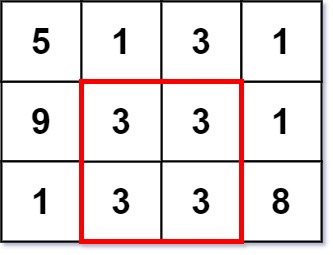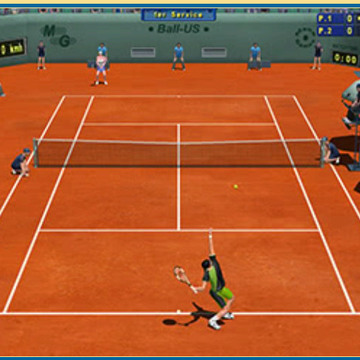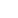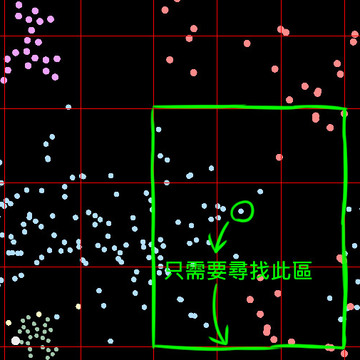# LeetCode - 1895. Largest Magic Square　解題心得

Not In My Back Yard | 2021-09-25 00:00:04 | 巴幣 2 | 人氣 31

m == grid.length
n == grid[i].length
1 ≦ m 、 n ≦ 50
1 ≦ grid[i][j] ≦ 10 ^ 6- 列之和： 5 + 1 + 6 = 5 + 4 + 3 = 2 + 7 + 3 = 12
- 行之和： 5 + 5 + 2 = 1 + 4 + 7 = 6 + 3 + 3 = 12
- 對角線和： 5 + 4 + 3 = 6 + 4 + 2 = 12### 相關創作Tennis Elbow 2013（TE 2013）遊戲初探09達人 Go 語言的陣列，搭配迴圈輕鬆省事28911《Unity練習》微生物 模擬小遊戲5166